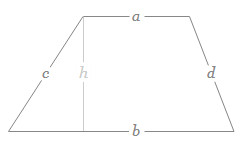## Trapezoid/Trapezium Area Calculator

 First parallel side of Trapezium Other parallel side of Trapezium Height of Trapezium Unit FeetInchesMeterCenti MeterMilli Meter
 Result: Area of Trapezium Unit

Trapezium Calculator to find out the Trapezium Area for the given inputs of height and two parallel side length.

### Trapezium Area FormulaTrapezium Area = ((a + b)/2) h

where, h is the height, a and b are the lengths of two parallel sides

Thinkcalculator.com provides you helpful and handy calculator resources.